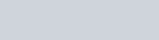The number of solid spheres,
Question:

The number of solid spheres, each of diameter 6 cm that could be moulded to form a solid metal cylinder of height 45 cm and diameter 4 cm, is

(a) 3

(b) 4

(c) 5

(d) 6

Solution:

Here,

Diameter of sphere = 6 cm

$=\frac{6}{2} \mathrm{~cm}$

$=3 \mathrm{~cm}$

Volume of the sphere

$=\frac{4}{3} \pi r^{3}$$=36 \pi$    $……..(i)$

Now,

Diameter of cylinder = 4 cm

Radius of cylinder $=\frac{4}{2} \mathrm{~cm}$

$=2 \mathrm{~cm}$

Height of the cylinder = 45 cm

Then,

Volume of the cylinder

$=\pi r^{2} h$

$=\pi \times 2 \times 2 \times 45$

$=180 \pi$

The number of solid sphere

$=\frac{\text { volume of cylinder }}{\text { volume of sphere }}$

$=5$

The number of solid sphere is 5.

Hence, the correct answer is choice (c).